Courses

## IIT JAM: Radioactivity: Radioactive Disintegration & Laws IIT JAM Notes | EduRev

The document Radioactivity: Radioactive Disintegration & Laws IIT JAM Notes | EduRev is a part of the IIT JAM Course Inorganic Chemistry.
All you need of IIT JAM at this link: IIT JAM

Radioactive decay (also known as nuclear decay or radioactivity) is the process by which an unstable atomic nucleus loses energy (in terms of mass in its rest frame) by emitting radiation.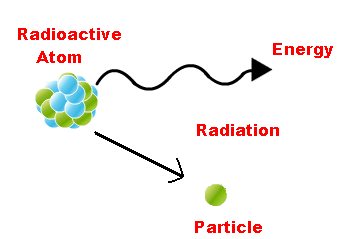• It looses energy by emitting radiations, such as an alpha particle, beta particle with neutrino or only a neutrino in the case of electron capture, gamma-ray, or electron in the case of internal conversion.
• A material containing such unstable nuclei is considered radioactive. Certain highly excited short-lived nuclear states can decay through neutron emission, or more rarely, proton emission.
• Radioactive decay is a stochastic (i.e. random) process at the level of a singular quantum of single atoms, in that, according to quantum theory, it is impossible to predict when a particular atom will decay, regardless of how long the atom has existed.
• However, for a collection of atoms, the collection's expected decay rate is characterized in terms of their measured decay constants or half-lives. This is the basis of radiometric dating.
• The half-lives of radioactive atoms have no known upper limit, spanning a time range of over 55 orders of magnitude, from nearly instantaneous to far longer than the age of the universe.
• All the heavy elements possess radioactive properties. However, the radioactive property of the different radioactive elements differs widely, e.g. radium atoms have about three million times the activity of uranium atoms.
• Uranium in the form of potassium uranyl sulphate, KUO2(SO4)2 was the first compound found to be radioactive. Radioactive changes are spontaneous. These are not controlled by temperature, pressure of nature of chemical combination.

The atomic nuclei of radioactive elements can disintegrate any moment. During disintegration, atoms of new elements having different physical and chemical properties are formed, called daughter elements.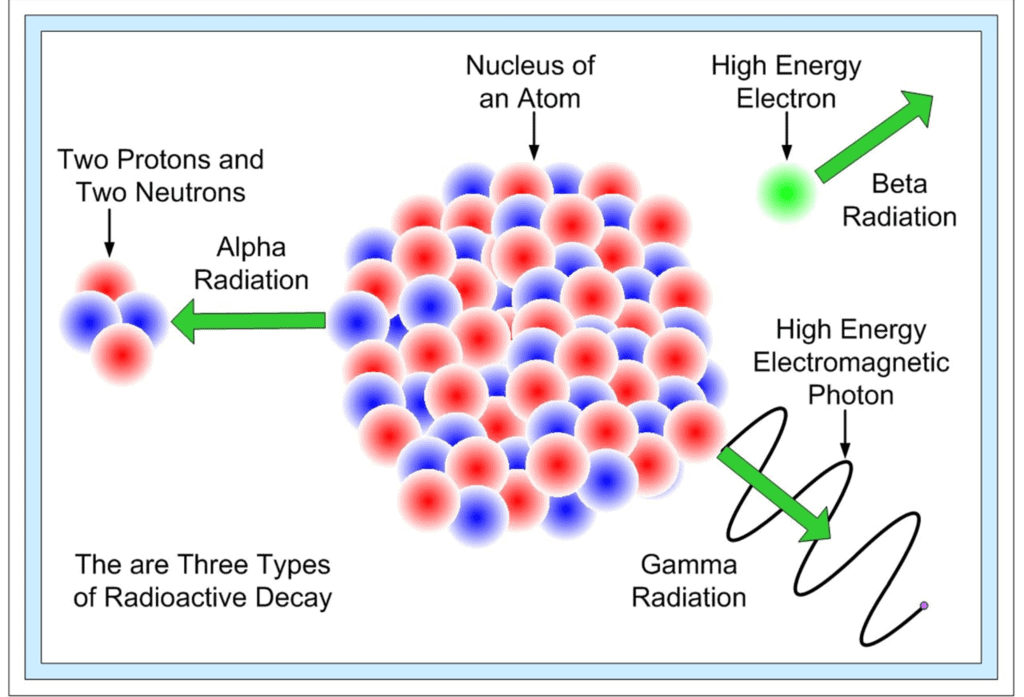Disintegration occurs by the following processes:

(i) α-particle emission:

When an α-particle  (4/2  He) is emitted from the nucleus of parent element, the new element formed called the daughter element, possess atomic mass less by 4 unit & atomic number less by 2 units.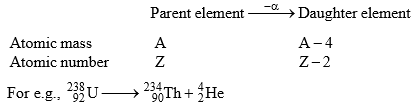(ii) β-particle emission:

When β-particle is emitted from parent element thus formed daughter elements possess possesses same atomic mass but atomic no. is increased by 1 unit.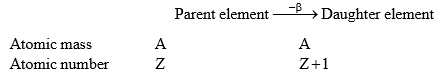•  Elements having same mass number called isobars.  (A-same, Z-different)

∴ daughter elements formed by the β-particle emission is an isobar of parent element.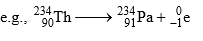If in a radioactive transformation α & β both are emitted then atomic mass & atomic number changes accordingly & produces an isotope of the parent element.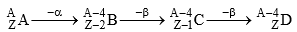(A & D) are isotopes)

Some Important Terms:

• Element having same no. of protons called Isotopes.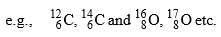(A-different, Z-different)
• Elements having same no. of neutrons are called Isotones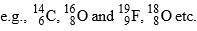(A-different, Z-different)
• Elements having same value of (A-2Z) are called Isodiaphers.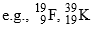(A-different, Z-different, A-2Z-same)
• Elements having same number of electrons are called Isoelectronic.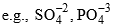• Compounds having same number of atoms as well as same number of electrons are called Isoster

The law of radioactive decay states that at any instant the rate of radioactive disintegration is directly proportional to the number of nuclei present in it, at that instance of time.

• The disintegration is at random, i.e., every atom has equal chance for disintegration any time.
• The number of atoms that disintegrate per second is directly proportional to the number of remaining unchanged radioactive atoms present at any time.
• The disintegration is independent of all physical and chemical conditions like temperature, pressure chemical combination etc. The two laws of radioactive disintegration can be summed up as below:

1. Group Displacement Law:

The result of α-and β-particle changes can be summed up in the form of group-displacement law. “In an α -particle change the resulting element has an atomic weightless by four units and atomic number less by two units and it falls in a group of the periodic table two columns to the left of the original element, and in a β-particle change the resulting element has same atomic weight but its atomic number is increased by one than its parent and hence it lies one column to right."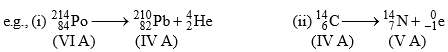According to the law of radioactive decay, the quantity of a radioelement that disappears in unit time (rate of disintegration) is directly proportional to the amount present.

Determination of the number of α-and β-particles emitted in a nuclear reaction:

Consider the following general reaction.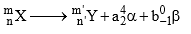Then, m = m’ + 4a + 0b (ii) n = n’ + 2a – b

Solve for a and b

Where a is the number of1He 4 emitted and b is the of-1β 0 emitted.

Decay Constant

The ratio between the number of atoms disintegrating in unit time to the total number of atoms present at that time is called the decay constant of that nuclide.

Characteristics of decay constant (λ)

1. It is characteristic of a nuclide (not of an element)

2. Its units are time–1

3. Its value is always less than one.

Points to Remember

1. Rate of decay (activity, A) is the number of atoms undergoing decay to unit time; it is represented by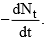2. Rate of decay of a nuclide is directly proportional to the number of atoms of that nuclide present at that moment, hence.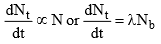(the negative sign shows that the number of radioactive atoms, Nt decreases as time t increases)

3. Rate of decay of nuclide is independent of temperature, so its energy of activation is zero.

4. Since the rate decay is directly proportional to the amount of the radioactive nuclide present and as the number of unrecompensed atoms decreases with increase in time, the rate of decay also decreases with the increase in time.

5. Various forms of equation for radioactive decay are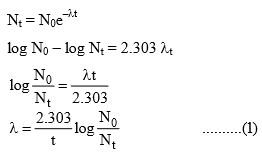Note that the equation (I) is similar to that of first-order reaction, hence we can say that radioactive disintegration are examples of first-order reactions.

Where N0 = Initial number of atoms of the gives nuclide, i.e. at time 0

Nt = No. of atoms of that nuclide present, i.e. at time 0

λ = Decay constant

However, unlike first-order rate constant (K), the decay constant (λ) is independent of temperature.

The document Radioactivity: Radioactive Disintegration & Laws IIT JAM Notes | EduRev is a part of the IIT JAM Course Inorganic Chemistry.
All you need of IIT JAM at this link: IIT JAMUse Code STAYHOME200 and get INR 200 additional OFF Use Coupon Code
All Tests, Videos & Notes of IIT JAM: IIT JAM## Inorganic Chemistry

49 videos|71 docs|16 tests

Track your progress, build streaks, highlight & save important lessons and more!

,

,

,

,

,

,

,

,

,

,

,

,

,

,

,

,

,

,

,

,

,

;